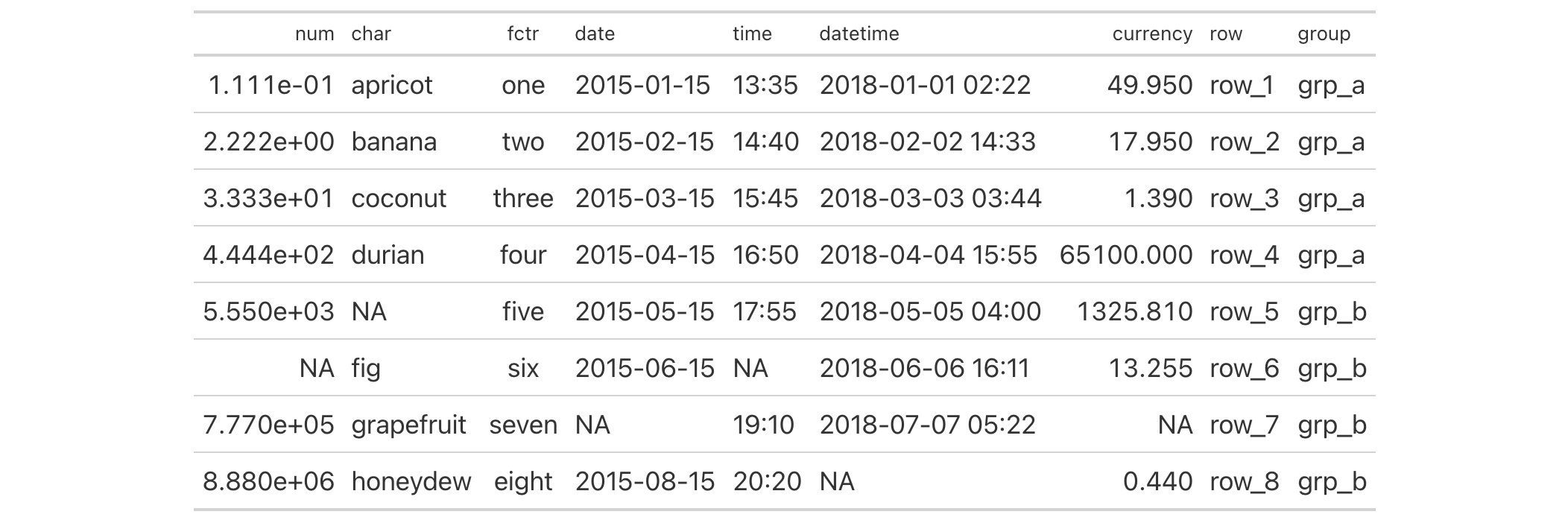A percentage value acts as a length value that is relative to an initial state. For instance an 80 percent value for something will size the target to 80 percent the size of its 'previous' value. This type of sizing is useful for sizing up or down a length value with an intuitive measure. This helper function can be used for the setting of font sizes (e.g., in cell_text()) and altering the thicknesses of lines (e.g., in cell_borders()). Should a more exact definition of size be required, the analogous helper function pct() will be more useful.

## Usage

pct(x)

## Arguments

x

Numeric value in percent

scalar<numeric|integer> --- required

The numeric value to format as a string percentage for some tab_options() arguments that can take percentage values (e.g., table.width).

## Value

A character vector with a single value in percentage units.

## Examples

Use the exibble dataset to create a gt table. Inside of the cell_text() call (which is itself inside of tab_style()), we'll use the pct() helper function to define the font size for the column labels as a percentage value.

exibble |>
gt() |>
tab_style(
style = cell_text(size = pct(75)),
locations = cells_column_labels()
)8-4

## Function Introduced

v0.2.0.5 (March 31, 2020)

Other helper functions: adjust_luminance(), cell_borders(), cell_fill(), cell_text(), cells_body(), cells_column_labels(), cells_column_spanners(), cells_footnotes(), cells_grand_summary(), cells_row_groups(), cells_source_notes(), cells_stub_grand_summary(), cells_stub_summary(), cells_stubhead(), cells_stub(), cells_summary(), cells_title(), currency(), default_fonts(), escape_latex(), google_font(), gt_latex_dependencies(), html(), md(), px(), random_id(), stub(), system_fonts()